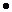Create a new printableMiddle School Math
Math Worksheets

Sample - Click above to make a new math worksheet (PDF).
 Name _____________________________Date ___________________
Measurement
Complete the unit conversions.

1.   3 centimeters to millimeters
3 cmmmcm
=    ________  millimeters
2.   9 centiliters to milliliters
9 cLmLcL
=    ________  milliliters
3.   288 inches to feet
288 inftin
=    ________  feet
4.   30 pints to quarts
30 ptqtpt
=    ________  quarts
5.   23 gallons to pints
23 galptgal
=    ________  pints
6.   200 centigrams to grams
200 cggcg
=    ________  grams
7.   6 pounds to ounces
6 lbozlb
=    ________  ounces
8.   240 hours to days
240 hoursdayshours
=    ________  days
 9 * This is a pre-made sheet.Use the link at the top of the page for a printable page.
10.   144 ounces to pounds
144 ozlboz
=    ________  pounds
11.   25 feet to inches
25 ftinft
=    ________  inches
12.   4 mins to secs
4 minssecsmins
=    ________  secs
13.   34 pints to quarts
34 ptqtpt
=    ________  quarts
14.   19 gallons to quarts
19 galqtgal
=    ________  quarts
15.   30 centiliters to milliliters
30 cLmLcL
=    ________  milliliters
16.   300 centigrams to grams
300 cggcg
=    ________  grams
17.   384 ounces to pounds
384 ozlboz
=    ________  pounds
18.   11 centiliters to milliliters
11 cLmLcL
=    ________  milliliters
 19 * This is a pre-made sheet.Use the link at the top of the page for a printable page.
20.   230 milligrams to centigrams
230 mgcgmg
=    ________  centigrams